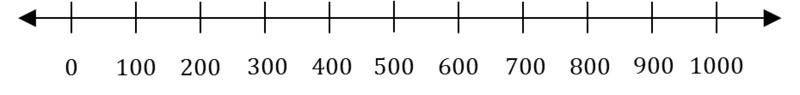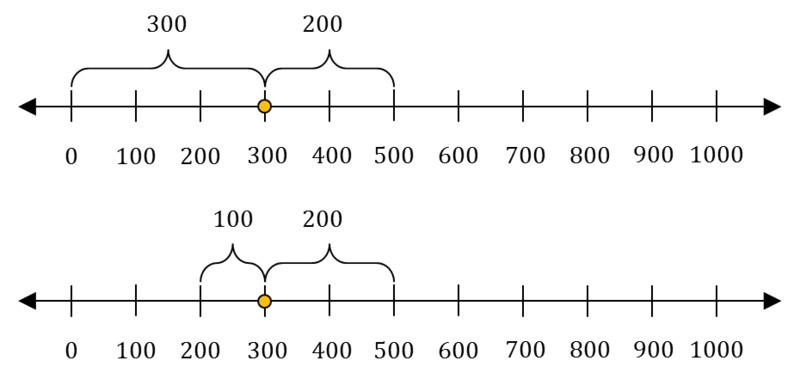# What's My Number?

Alignments to Content Standards: 4.NBT.A

1. Find a number greater than 0 and less than 1,000 that:

• Is closer to 500 than 0,
• and

• Is closer to 200 than 500.2. There are many correct answers to this problem. Describe all of the numbers that are correct.

## IM Commentary

The purpose of this task is for students to reason about base-ten numbers on the number line in a way that will require them to use what they know about place value. Students first represent addition and subtraction on the number line in grade 2 (see 2.MD.6). The standards explicitly call on students to use number lines to solve problems involving fractions and time in third grade (see 3.NF.A and 3.MD.1) and decimals and measurement quantities in 4th grade (see 4.NF.6 and 4.MD.2). The number line is also a very powerful tool for helping students understand place value, and students should be encouraged to use it in third and fourth grade to support their deepening understanding of base-ten numbers. This task provides students such an opportunity, and as such, is aligned to the cluster 4.NBT.A, Generalize place value understanding for multi-digit whole numbers.

Depending on students' comfort with the number line and place value, the teacher could choose to discuss the task in three parts: identifying numbers between 0 and 1000 that are closer to 500 than to 0; identifying numbers between 0 and 1000 that are closer to 200 than 500, and, finally, identifying the numbers that are common to both. Each of these three parts can be further divided into (1) separating numbers that fit the description from numbers that do not fit the description to produce a correct response and (2) describing all correct answers. Teachers might consider having the students work on part (a) and then working together as a class to tackle part (b), giving the class a similar problem for independent or group work. Note that while the task doesn't explicitly ask students to use symbolic notation for "greater than" and "less than," the teacher should show the students how to do this if they don't do it on their own (see the solution for an example).

The following type of questions can be used to guide students through the task.

• Orient students to the number line: “What does 'greater than' mean on the number line?” (to the right of) “What does 'less than' mean on the number line?” (to the left of) “Our mystery number is greater than 0 and less than 1000. What is the smallest whole number that fits these clues?” (1) “What is the largest whole number that fits these clues?” (999) “Name some other numbers that are greater than 0 and less than 1000.” (The teacher plots 4 or 5 numbers that students volunteer on a number line visible to all) “What number is half-way between 0 and 1,000?” (500)
• Identify the set of numbers that are closer to 500 than to 0: "The second clue we have is that the number is closer to 500 than to 0. Who thinks they know a number that fits this second clue? (any number between 250 and 1000) Teacher plots all suggestions, even those that are incorrect, and discusses each one. “Why do you think that 650 is closer to 500 than 0?” (shorter distance) “Why do you think 300 is closer to 500 than 0?” “What about 150? Is it closer to 500 or 0?” (0) The teacher continues discussing numbers that approach 250 from both directions until it is not possible to be sure by looking whether a number is closer to 0 or to 500. “What number is in the exact middle between 0 and 500?” (250) “How do you know? So what can we say about all of the numbers that are greater than 250? Less than 250?” The discussion should continue until students generalize that all of the numbers to the right of 250 are closer to 500 and that all of the numbers to the left of 250 are closer to 0. The teacher identifies that section of the number line.
• Identify the set of numbers that are closer to 200 than to 500: ”The third clue we have is that the number is closer to 200 than to 500.” Through a discussion similar to above, students should be led to the generalization that all of the numbers to the left of 350 are closer to 200, while all of the numbers to the right of 350 are closer to 500. The set is identified on the class number line.
• Identify the set of numbers common to both clues: ”Who has a number that fits both clues?” (any number greater than 250 and less than 350) “How do you know? Should 250 be included?" (no) "Why not? (250 is exactly in the middle and exactly the same distance from 0 as it is from 500.) "Should 350 be included?" (no) "Why not?" (250 is exactly in the middle and exactly the same distance from 0 as it is from 500.)

## Solution

1. One example of many is 300. To see this, note that 500 - 300 = 200, so 300 is closer to 500 than 0. Also, 300 - 200 = 100, so 300 is closer to 200 than 500.2. 250 is half-way between 0 and 500. Any number greater than 250 will be closer to 500 than it is to 0.

350 is half-way between 200 and 500. Any number less than 350 will be closer to 200 than it is to 500.

We are looking for numbers that satisfy both of the above conditions. Thus, the answer is all numbers that are greater than 250 and less than 350.Note that $300 \gt 250$ and $300 \lt 350$, so this is consistent with our example in part (a).